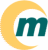s i s t e m a   o p e r a c i o n a l   m a g n u x   l i n u x ~/ · documentaçăo · suporte · sobre

Next Previous Contents

## 4. Pipes

This section explains in a very simple and practical way how to use pipes, nd why you may want it.

## 4.1 What they are and why you'll want to use them

Pipes let you use (very simple, I insist) the output of a program as the input of another one

## 4.2 Sample: simple pipe with sed

This is very simple way to use pipes.

``` ls -l | sed -e "s/[aeio]/u/g" ```
Here, the following happens: first the command ls -l is executed, and it's output, instead of being printed, is sent (piped) to the sed program, which in turn, prints what it has to.

## 4.3 Sample: an alternative to ls -l *.txt

Probably, this is a more difficult way to do ls -l *.txt, but it is here for illustrating pipes, not for solving such listing dilema.

``` ls -l | grep "\.txt\$" ```
Here, the output of the program ls -l is sent to the grep program, which, in turn, will print lines which match the regex "\.txt\$".
Next Previous Contents